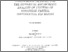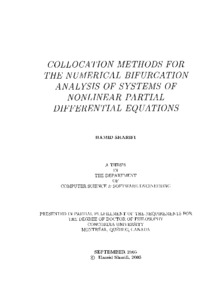Title:

# Collocation methods for the numerical bifurcation analysis of systems of nonlinear partial differential equations

Sharifi, Hamid (2005) Collocation methods for the numerical bifurcation analysis of systems of nonlinear partial differential equations. PhD thesis, Concordia University.Preview Text (application/pdf) NR09969.pdf - Accepted Version 8MB

## Abstract

The study of nonlinear phenomena has been an important endeavor for scientists. Some nonlinear phenomena can be modeled mathematically as nonlinear partial differential equations (PDEs). There are no analytical solutions for most nonlinear PDEs. Therefore, an appropriate numerical method must be used in order to compute an adequate approximate solution. A new class of numerical methods, called Finite Element Collocation Methods with Discontinuous Piecewise Polynomials, has recently been proposed for solving nonlinear elliptic PDE. In this thesis, this method has been generalized for solving nonlinear elliptic PDE systems using an alternative nested dissection solution procedure. Using a modified formulation of the pseudo-arclength continuation method, we have used this method in continuation studies and in the numerical bifurcation analysis of nonlinear PDE systems. In the thesis the method is introduced gradually, starting with the simplest case, linear ODE BVPs, followed by nonlinear ODE BVPs, linear scalar PDEs, nonlinear scalar PDEs, continuation problems in nonlinear scalar PDEs, and, finally, continuation problems for systems of nonlinear PDEs. AUTO has probably been the most widely used continuation software package for ODE problems. The collocation method introduced in this thesis, as well as the numerical method used to solve the resulting systems of nonlinear equations, can be viewed as a generalization to PDEs of the robust and powerful techniques for ODE BVPs that have made AUTO so widely used in computations and as a model for other continuation software projects. As a part of the research toward the construction of an AUTO-like software package for PDE problems, prototype software has been developed for the numerical bifurcation analysis of nonlinear elliptic PDE systems in two-dimensional space (2D). The UML (The Unified Modelling Language) notation is used to present the implementation algorithms and our object-oriented prototype software. We consider several test problems, as well as some practical applications, such as the Bratu-Gelfand problem, the Brusselator system, and the streamfunction-vorticity formulation of the Navier-Stokes equations for a two-dimensional incompressible fluid flow problem. These examples demonstrate the capabilities and the strength of the collocation method with discontinuous elements for solving substantial PDEs continuation problems.

Divisions: Concordia University > Gina Cody School of Engineering and Computer Science > Computer Science and Software Engineering Thesis (PhD) Sharifi, Hamid xvii, 226 leaves : ill. (some col.) ; 29 cm. Concordia University Ph. D. Computer Science and Software Engineering 2005 Doedel, Eusebius LE 3 C66C67P 2005 S53 8551 Concordia University Library 18 Aug 2011 18:28 13 Jul 2020 20:04 https://concordiauniversity.on.worldcat....
All items in Spectrum are protected by copyright, with all rights reserved. The use of items is governed by Spectrum's terms of access.

Repository Staff Only: item control pageResearch related to the current document (at the CORE website)
Back to top